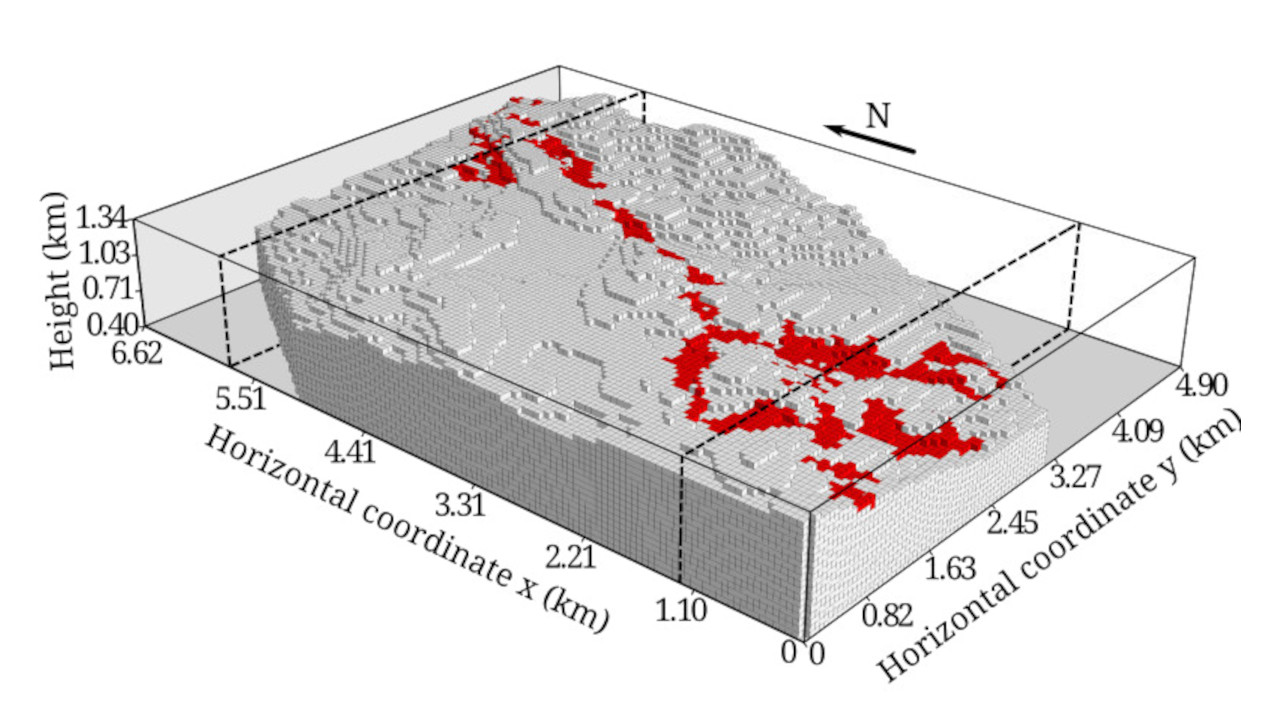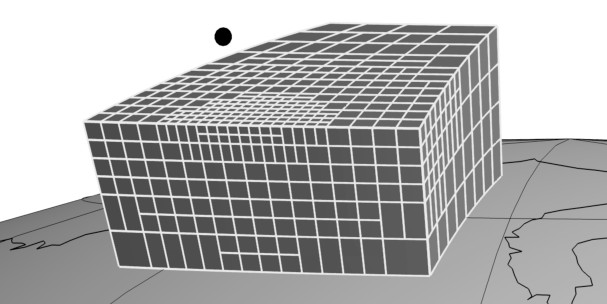In the Computer-Oriented Geoscience Lab we create and use open-source software to undertake research into geophysical inversion, modelling, and data processing.

## Inverse problems

Our ultimate goal as geophysicists is to infer the physical properties of the inner Earth and its processes from surface observations. This is an ill-posed inverse problem, to which a solution might not exist or be non-unique and unstable. We develop methods for solving different kinds of inverse problems using several sets of constraints to overcome the instability of the solution.Distribution of an iron ore deposit in Brazil estimated from gravity gradient data.

## Forward modeling

A key component for solving an inverse problem is first solve the "forward problem". This is jargon for predicting data given a set of model parameters. One of the main research problems on which we work is developing a method for forward modeling gravitational fields caused by a tesseroid (a spherical prism).A tesseroid discretized using our adaptive algorithm.

## Data processing

There is no turning back from the machine learning frenzy that has taken over the world. Geoscientists have been doing similar things for decades but with different names and objectives. One of these things is called the "equivalent layer technique" in gravity and magnetics. Similar methods in different fields have many different names, for example radial basis functions or Green's functions interpolation. All of these methods are linear regressions in which we fit a linear model to some data and then use the model to predict new data. Given the many similarities, we are applying other machine learning techniques to these geophysical problems.Spatial data has uncertainties which need to be handled properly. There are different ways to use uncertainties as data weights for different algorithms, like interpolation and blocked statistics.